Abstract: 数字图像处理：第46天
Keywords: canny,边缘检测

# 灰度图像-图像分割 Canny边缘检测

Canny是目前已知的最好的边缘检测算法，是不是之一我不确定，但可以肯定的是，它的应用非常广泛，基本用到边缘检测的，大家永远第一个想到它。Canny算法的复杂度比前面的检测加阈值的算法计算复杂度更高，空间复杂度也要高一些，但现在的计算设备，对于Canny基本可以实现实时，并且有人用GPU来实现，所以从86年Canny提出了这个算法到现在，在边缘检测方面，其地位还是比较稳固的。
Canny算法的另一个显著特征是它有完整的数学推导过程，能够证明这个算法能给出最好的边缘。后面我们将会简单的看一下数学过程。

## 算法原理

1) Good detection. There should be a low probability of failing to mark real edge points, and low probability of falsely marking nonedge points. Since both these probabilities are monotonically decreasing functions of the output signal-to-noise ratio, this criterion corresponds to maximizing signal-to-noise ratio.
2) Good localization. The points marked as edge points by the operator should be as close as possible to the center of the true edge.
3) Only one response to a single edge. This is implicitly captured in the first criterion since when there are two responses to the same edge, one of them must be considered false. However, the mathematical form of the first criterion did not capture the multiple response requirement and it had to be made explicit.

1. 好的检测：一定要尽可能少的遗漏边缘点，尽可能少的添加非边缘点。正确的边缘点为信号，被错误检测出来的非边缘点为噪声，所以第一点归结为提高信噪比。
2. 准确的位置：检测出的边缘点一定要与真正的边缘中心，尽可能的近。
3. 边缘单一响应：对于一个边缘，只能产生一个响应，如果对于一个边缘产生两个响应，第一点的数学求解过程不能保证这一点，所以要单独明确，如果对于一边有两个响应，必须去掉一个。

## 数学原理

$H_G(x)=\int_{-w}^{+w} G(-x)f(x)\,dx$ (1)

$H_n=n_0[\int_{-w}^{+w}f^2(x)\,dx]^{1/2}$ (2)

$SNR=\frac{\int_{-w}^{+w} G(-x)f(x)\,dx}{n_0[\int_{-w}^{+w}f^2(x)\,dx]^{1/2}}$ (3)

$H^{‘}_n(x_0)+H^{‘}_G(x_0)=0$ (4)

$H^{‘}_G(x_0)=H^{‘}_G(0)+H^{‘’}_G(0)x_0+o(x_0^2)$ (5)

$H^{‘’}_G(0)x_0 \approx {-H^{‘}_n(x_0)}$(6)

$H^{‘}_n(x_0)$ 为高斯随机量，并且其方差是 $H^{‘}_n(x_0)$ 的均方值，并且给出其期望为：

$E[H^{‘}_n(x_0)^2]=n_0^2\int^{+w}_{-w}f^{‘2}(x)\,dx$(7)

$E[x^2_0]\approx {\frac{n_0^2\int^{+w}_{-w}f^{‘2}(x)\,dx}{[{\int_{-w}^{+w} G^{‘}(-x)f^{‘}(x)\,dx}]^2}}=\delta x^2_0$ (8)

$\delta x^2_0$ 是 $x_0$ 标准差的近似，位置由其倒数给出：

$Localization =\frac{|\int^{+w}_{-w}G^{‘}(-x)f^{‘}(x)\,dx|}{n_0[\int^{+w}_{-w}f^{‘2}(x)\,dx]^{-1/2}}$(9)

$\frac{\int_{-w}^{+w} G(-x)f(x)\,dx}{n_0[\int_{-w}^{+w}f^2(x)\,dx]^{1/2}}\frac{|\int^{+w}_{-w}G^{‘}(-x)f^{‘}(x)\,dx|}{n_0[\int^{+w}_{-w}f^{‘2}(x)\,dx]^{-1/2}}$(10)

$n^{-1}_0[\int^{+w}_{-w}G^{2}(x)dx]^{1/2}$

Localization(9)上边界于：

$n^{-1}_0[\int^{+w}_{-w}G’^{2}(x)dx]^{1/2}$

$x_{ave}=\pi(\frac{-R(0)}{R^{‘’}(0)})^{1/2}$(11)

$R(0) = \int ^{+\infty}_{-\infty}g^{2}(x)\,dx$

$R^{‘’}(0) = -\int ^{+\infty}_{-\infty}{g’}^{2}(x)\,dx$

$x_{zc}(f)=\pi(\frac{\int ^{+\infty}_{-\infty}f’^{2}(x)\,dx}{\int ^{+\infty}_{-\infty}{f’’}^{2}(x)\,dx})^{1/2}$(12)

$x_{max}(f)=2x_{zc}(f)=kW$(13)

$N_n=\frac{2W}{x_{max}}=\frac{2}{k}$(14)

## 算法过程

1. 高斯平滑，采用$5 \times5$的高斯滤波器对图像进行平滑。
2. 使用Sobel算子检测边缘候选点，计算梯度方向，得到简化的梯度方向。
3. 非极大值抑制，减少多重响应。
4. 边缘跟踪，采用双阈值处理候选点。
5. 形态学细化，对于有些较粗的边界采用形态学方法处理。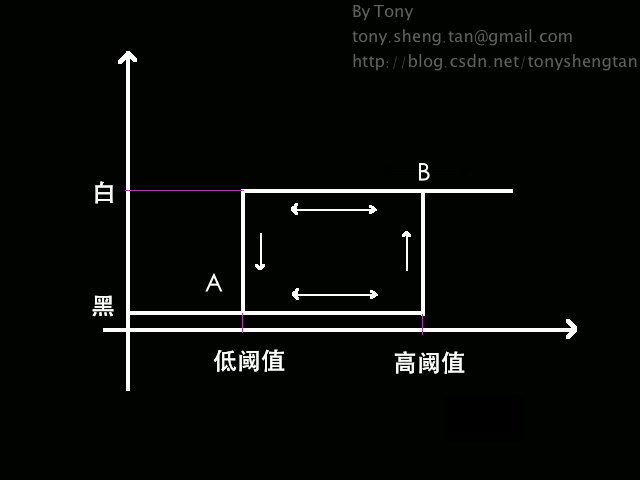## 实现结果STEP1：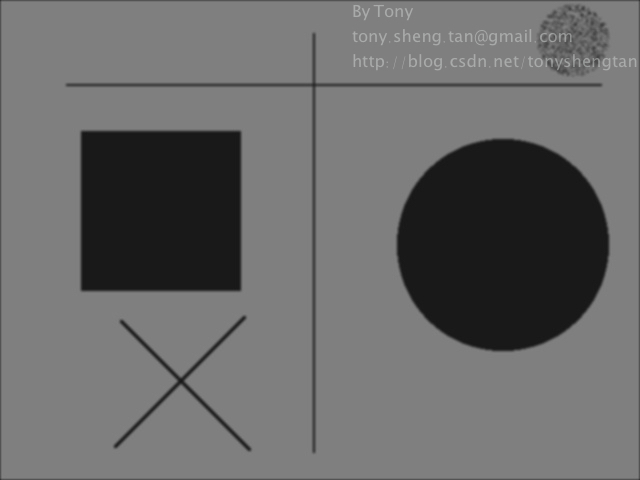STEP2：
Sobel梯度幅度结果：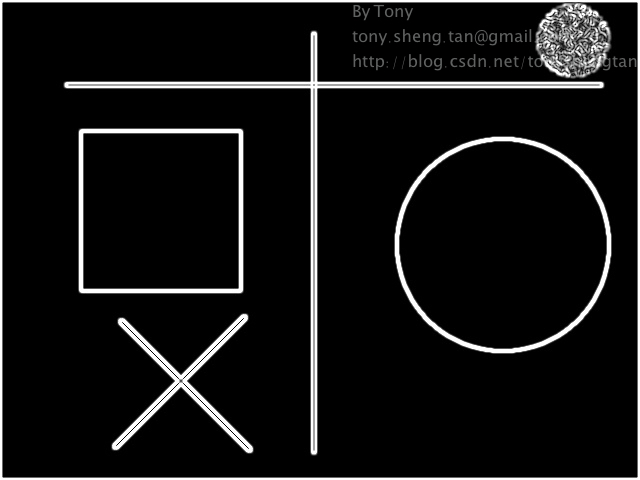Sobel梯度方向结果：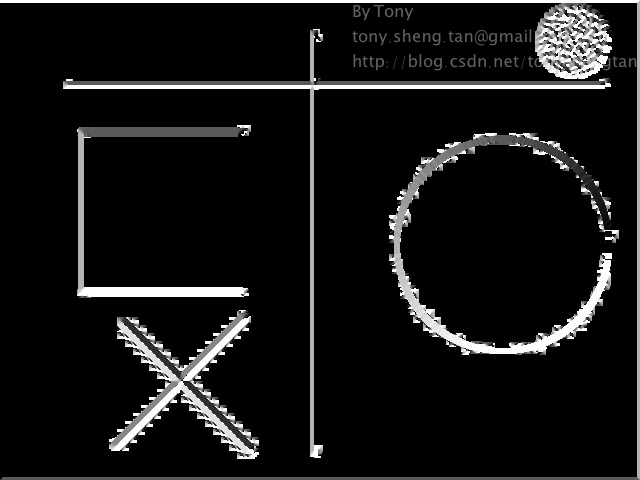STEP3：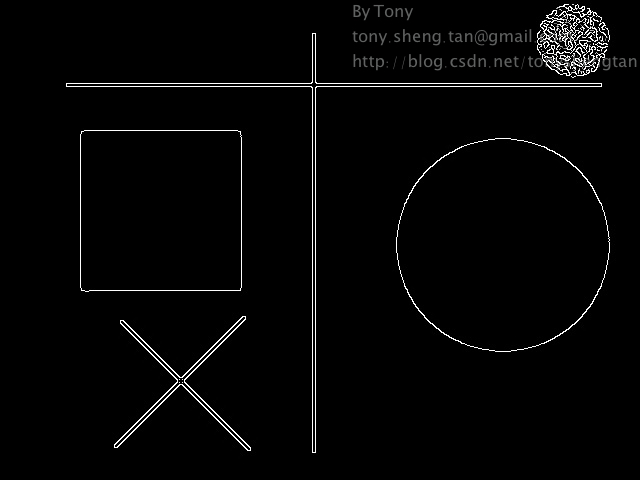STEP4：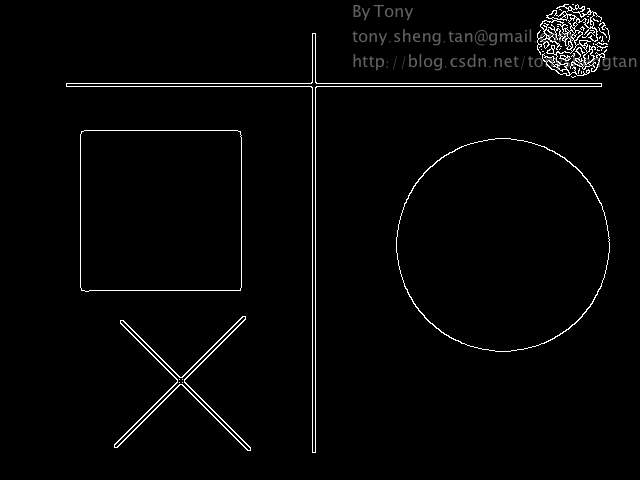STEP1：STEP2：
Sobel梯度幅度结果：Sobel梯度方向结果：STEP3：STEP4：0%